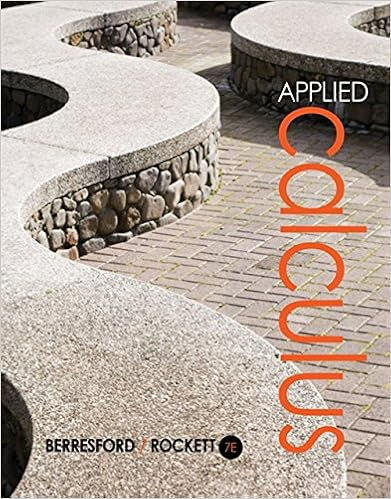# Completing the square x 2 bx c x b 2 2 c b 2 4

• Homework Help
• 65
• 60% (5) 3 out of 5 people found this document helpful

This preview shows page 13 - 16 out of 65 pages.

##### We have textbook solutions for you!
The document you are viewing contains questions related to this textbook.The document you are viewing contains questions related to this textbook.
Chapter 5 / Exercise 80
Applied Calculus
Berresford/RockettExpert Verified
Completing The Square : x 2 + bx + c = ( x + b 2 ) 2 + ( c – b 2 4 ) . Practice 1 : Evaluate 5 x 2 + 8x + 25 dx by completing the square and changing the variable. Example 2 : Evaluate 6x x 2 – 6x + 10 dx . Solution: This would be an easy problem if the numerator was 6x – 18. Then the numerator would be three times the derivative of the denominator and the pattern of the integral would be 3 u du with u = x 2 – 6x + 10. Fortunately, we can rewrite the numerator as 6x – 18 + 18. Then 6x x 2 – 6x + 10 dx = 6x – 18 + 18 x 2 – 6x + 10 dx = 6x – 18 x 2 – 6x + 10 dx + 18 x 2 – 6x + 10 dx = 3 u du + 18 w 2 + 1 dw where u = x 2 – 6x + 10 and w = x – 3 = 3 . ln| u | + 18 . arctan( w ) + C = 3 . ln( x 2 – 6x + 10 ) + 18 . arctan( x – 3 ) + C .
##### We have textbook solutions for you!
The document you are viewing contains questions related to this textbook.The document you are viewing contains questions related to this textbook.
Chapter 5 / Exercise 80
Applied Calculus
Berresford/RockettExpert Verified
8.2 Integration Review Contemporary Calculus 3 Practice 2 : Evaluate 4x + 21 x 2 + 8x + 25 dx . (Hint: if u = x 2 + 8x + 25 , then 2 du = (4x + 16) dx .) The "logarithm plus an arctangent" pattern of these answers is quite typical for integrals of linear functions divided by irreducible quadratics. If the quadratic denominator can be factored into a product of two linear factors, we use the technique discussed in Section 8.3, Partial Fraction Decomposition. Problems In problems 1 – 42, find the indefinite integrals and evaluate the definite integrals. In some of the problems a particular change of variable is suggested. 1. 6 x ( x 2 + 7) 2 dx u = x 2 + 7 2. 6 x ( x 2 – 1) 3 dx u = x 2 – 1 3. 2 4 6 x x 2 – 3 dx u = x 2 – 3 4. 0 π 12 cos( x ) . {sin( x ) + 2} 2 dx u = sin( x ) + 2 5. 12 x x 2 + 3 dx 6. cos( x ) 2 + sin( x ) dx 7. sin(3 x + 2) dx 8. cos( x /5 ) dx 9. 0 1 e x sec 2 ( e x + 3) dx u = e x + 3 10. 0 π /2 cos( x ) 1 + sin( x ) dx u = 1 + sin( x ) 11. ln( x ) x dx u = ln( x ) 12. cos( x ) x dx u = x 13. cos( x ) . e sin( x ) dx 14. e x sin( e x ) dx 15. 1 3 5 1 + 9 x 2 dx u = 3 x 16. 0 1 7 1 + ( x + 3) 2 dx u = x + 3 17. 1 2 1 x 2 cos( 1 x ) dx u = 1 x 18. 1 e sec(2 + ln( x )) x dx u = 2 + ln( x ) 19. 6 sin( x ) cos( x ) 5 + sin 2 ( x ) dx u = 5 + sin 2 ( x ) 20. 6 cos( x ) 5 + sin 2 ( x ) dx u = sin( x ) 21. 10 2 x + 5 dx 22. 3 8 x + 1 dx
8.2 Integration Review Contemporary Calculus 4 23. 1 3 20 x 5 x 2 + 3 dx 24. 1 5 4 x x 2 + 9 dx 25. 0 1 7 ( x + 3) 2 + 4 dx u = x + 3 26. –2.1 –2.3 1 1 – ( x + 2) 2 dx u = x + 2 27. e x 1 + e 2 x dx 28. 4 x + 10 x 2 + 5 x + 9 dx u = x 2 + 5 x + 9 29. 1 e 3 x . { 1 + ln( x ) } dx 30. 0 1 e x 1 + e x dx 31. 0 1 2 x 1 – x 2 dx 32. 0 3 2 x 5 + x 2 dx 33. cos( x ) (1 + sin( x ) ) 3 dx 34. cos( x ) sin 4 ( x ) dx 35. 1 e ln( x ) x dx 36. 1 2 e x 2 + e x dx 37. sec 2 ( x ) 5 + tan( x ) dx 38. 6 x ( x 2 – 1) 3 dx 39. tan( x – 5) dx 40. (x 3 + 3) 2 dx 41. 0 1 e 5 x dx 42. sec(2 + 3x) dx In problems 43 – 48, complete the square in the denominator, make the appropriate substitution, and integrate.
•••